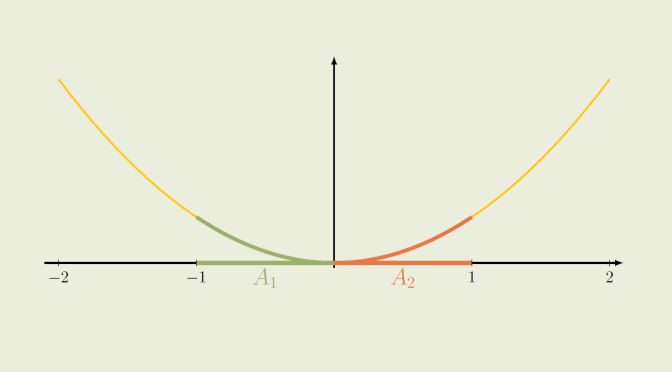# Playing with images and inverse images

We’re looking here to relational expressions involving image and inverse image.

We consider a function $$f : X \to Y$$ from the set $$X$$ to the set $$Y$$. If $$x$$ is a member of $$X$$, $$f(x)$$ is the image of $$x$$ under $$f$$.
The image of a subset $$A \subset X$$ under $$f$$ is the subset (of $$Y$$) $f(A)\stackrel{def}{=} \{f(x) : x \in A\}.$

The inverse image of a subset $$B \subset Y$$ is the subset of $$A$$ $f^{-1}(B)\stackrel{def}{=} \{x \in X : f(x) \in B\}.$ Important to understand is that here, $$f^{-1}$$ is not the inverse function of $$f$$.

We now look at relational expressions involving the image and the inverse image under $$f$$.

### Inverse images with unions and intersections

Following relations hold:
$\begin{array}{c} f^{-1}(B_1 \cup B_2) = f^{-1}(B_1) \cup f^{-1}(B_2)\\ f^{-1}(B_1 \cap B_2) = f^{-1}(B_1) \cap f^{-1}(B_2) \end{array}$ Let’s prove the first equality as an example. We have $$x \in f^{-1}(B_1 \cup B_2)$$ if and only if $$f(x) \in B_1 \cup B_2$$ if and only if $$f(x) \in B_1$$ or $$f(x) \in B_2$$ which means exactly $$x \in f^{-1}(B_1) \cup f^{-1}(B_2)$$.

### Images with unions and intersections

Regarding images, we have following relations:
$\begin{array}{c} f(A_1 \cup A_2) = f(A_1) \cup f(A_2)\\ f(A_1 \cap A_2) \subset f(A_1) \cap f(A_2) \end{array}$ You can observe that in the second relation, we only have an inclusion and it is on purpose. The equality $$f(A_1 \cap A_2) = f(A_1) \cap f(A_2)$$ holds when $$f$$ is one-to-one. Let’s prove that $$f(A_1) \cap f(A_2) \subset f(A_1 \cap A_2)$$ in that case. $$y \in f(A_1) \cap f(A_2)$$ means that $$y \in f(A_1)$$ and $$y \in f(A_2)$$. Therefore, there exist $$x_1 \in A_1$$ and $$x_2 \in A_2$$ such that $$y=f(x_1)=f(x_2)$$. As $$f$$ is supposed to be one-to-one, we must have $$x_1 = x_2 \in A_1 \cap A_2$$. Consequently $$y=f(x_1)=f(x_2) \in f(A_1 \cap A_2)$$ which allows us to conclude.

However, $$f(A_1 \cap A_2)$$ can be a proper subset of $$f(A_1) \cap f(A_2)$$ when $$f$$ is not one-to-one and we provide a counterexample. Consider the real function $$f : x \mapsto x^2$$ and the subsets $$A_1 = [-1,0] \subset \mathbb R$$, $$A_2 = [0,1] \subset \mathbb R$$. We have $\begin{array}{l} A_1 \cap A_2 = \{0\} \text{ hence } f(A_1 \cap A_2) = \{0\} \\ f([-1,0]) = f([0,1])= [0,1] \text{ hence } f(A_1) \cap f(A_2) = [0,1] \end{array}$ we finally get $$f(A_1 \cap A_2) \varsubsetneq f(A_1) \cap f(A_2)$$ for that example.

### About the inclusions $$f(f^{-1}(B)) \subset B$$ and $$A \subset f^{-1}(f(A))$$

One can prove following inclusions:
$\begin{array}{c} f(f^{-1}(B)) \subset B\\ A \subset f^{-1}(f(A)) \end{array}$ The inclusion $$f(f^{-1}(B)) \subset B$$ becomes an equality if $$f$$ is onto as $$B \subset f(f^{-1}(B))$$ in that case. For the prove, consider $$y \in B$$. As $$f$$ is supposed to be onto, it exists $$x \in X$$ such that $$y=f(x)$$, which means that $$x \in f^{-1}(B)$$ and finally $$y=f(x) \in f(f^{-1}(B))$$. If $$f$$ is not onto, the inclusion $$f(f^{-1}(B)) \subset B$$ can be proper. Let’s consider the $$\sin$$ function and the subset $$B = [-1,2]$$. As $$B$$ contains the image of $$\sin$$, namely $$[-1,1]$$, we have $$f^{-1}([-1,2]) = \mathbb R$$ and $[-1,1]=f(f^{-1}([-1,2]))=f(f^{-1}(B)) \varsubsetneq B = [-1,2]$

For its part, the inclusion $$A \subset f^{-1}(f(A))$$ becomes an equality if $$f$$ is one-to-one. The proof is left to the reader. The inclusion can be proper if $$f$$ is not one-to-one. Consider again the map $$f : x \mapsto x^2$$ and $$A=[0,1]$$. We have $$f(A)=f([0,1])=[0,1]$$, $$f^{-1}(f(A)) = f^{-1}([0,1])=[-1,1]$$ and finally $$A \varsubsetneq f^{-1}(f(A))$$ as desired.